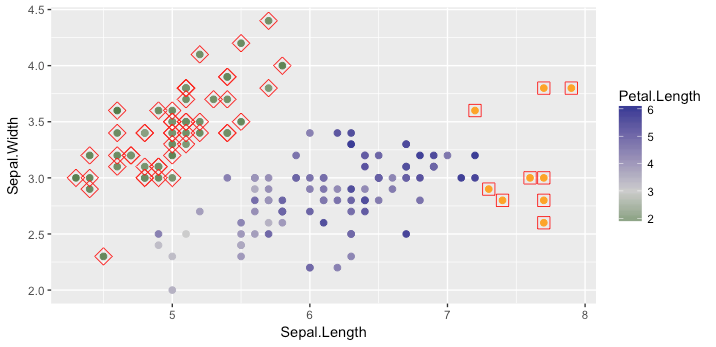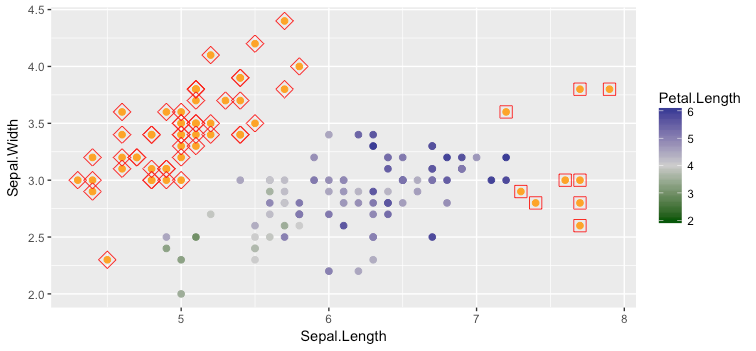Skip to content
{{ message }}

# tidyverse / ggplot2

Have a question about this project? Sign up for a free GitHub account to open an issue and contact its maintainers and the community.

By clicking “Sign up for GitHub”, you agree to our terms of service and privacy statement. We’ll occasionally send you account related emails.

Already on GitHub? Sign in to your account

# scale_color_gradient2 doesn't entirely respect limits argument #2230

Closed
opened this issue Aug 3, 2017 · 3 comments
Closed

# scale_color_gradient2 doesn't entirely respect limits argument#2230

opened this issue Aug 3, 2017 · 3 comments
Labels

## Comments

###rpruim commented Aug 3, 2017

 If `midpoint` is not half-way between the two values in `limits`, then the gradient scale extends beyond the limit that is closer to `midpoint` but the guide stops where `limits` specifies. Especially in conjunction with `na.value`, this can mislead the reader. In this plot the color scale goes below what the guide displays, and values below 2 are not indicated as `NA`. ```library(scales) library(ggplot2) library(dplyr) ggplot(mapping = aes(x = Sepal.Length, y = Sepal.Width) ) + geom_point(data = iris, aes(color = Petal.Length), size = 2) + geom_point(data = iris %>% filter(Petal.Length < 2), size = 4, shape = 5, color = "red") + geom_point(data = iris %>% filter(Petal.Length > 6), size = 4, shape = 0, color = "red") + scale_color_gradient2(low = muted("green"), high = muted("navy"), mid = "gray80", midpoint = 3, limits = c(2, 6), na.value = "orange")``` If we change `midpoint` to `3`, then the scale truly runs from 2 to 6 and values below 2 are flagged. ```ggplot(mapping = aes(x = Sepal.Length, y = Sepal.Width) ) + geom_point(data = iris, aes(color = Petal.Length), size = 2) + geom_point(data = iris %>% filter(Petal.Length < 2), size = 4, shape = 5, color = "red") + geom_point(data = iris %>% filter(Petal.Length > 6), size = 4, shape = 0, color = "red") + scale_color_gradient2(low = muted("green"), high = muted("blue"), mid = "gray80", midpoint = 4, limits = c(2, 6), na.value = "orange")``` The text was updated successfully, but these errors were encountered:

###karawoo commented Aug 3, 2017

 Thanks for opening this issue and including an example. Would you mind including the plot images as well? The reprex package can help streamline this.

###rpruim commented Aug 3, 2017

 Here you go. Notice the color change for `NA`'s when we switch the value of `midpoint` from plot 1 to plot 2. Things in diamonds or squares should be marked as `NA` (orange) if `limits` is being respected tightly.###foo-bar-baz-qux commented Aug 8, 2017

 I think the issue is to do with the `mid_rescaler` which calls `scales::rescale_mid`. If the midpoint is set correctly to be the middle of the limits, the scaled limits will be [0, 1] such that all points outside the original limits, would also be outside [0, 1]. This leads to the expected result of those points outside the range being marked as NA. However, the `midpoint` parameter might not do what is expected here if it is not in the middle of the data; if you look at the `scales::rescale_mid` function, it effectively changes the range of [0, 1] to correspond to twice the distance from the `midpoint` to either the minimum or maximum limit (whichever distance is greater). So using a `midpoint` of 3 instead of 4 in the original example, effectively scales the range [0, 6] down to [0, 1], rather than [2, 6] down to [0, 1] as one might expect. This leads to all points with `Petal.Length < 2` to still be displayed since all original petal lengths are > 0, and therefore will still be greater than 0 after the scaling. If you set the `midpoint` to 10, you get the opposite effect since now 10 is further from the minimum of the range (2) compared to the maximum of the range (6), so all points with petal length < 2 are considered NA since they will be < 0 in the scaled range. Some possible solutions (from least to most conservative): Set the default mid-point as being the middle of the `from` parameter in the function returned by `mid_rescaler`. The default of `midpoint = 0` can definitely lead to strange results depending on the range of the data. E.g. if the range of the data is 100 - 200, then a 0 midpoint effectively renders the `low` parameter unused. Throw a warning in `mid_rescaler` when mid-point is not in the middle of the range. Documentation change to reflect behavior when not actually set to the middle of the data range. Perhaps there's a use-case for manually setting the mid-point, but the current behaviour may not be obvious from the documentation
to subscribe to this conversation on GitHub. Already have an account? Sign in.
Projects
None yet
Linked pull requests

Successfully merging a pull request may close this issue.

None yet